Courses

# RD Sharma Solutions Ex-8.4, (Part -1), Lines And Angles, Class 9, Maths Class 9 Notes | EduRev

## Class 9 : RD Sharma Solutions Ex-8.4, (Part -1), Lines And Angles, Class 9, Maths Class 9 Notes | EduRev

The document RD Sharma Solutions Ex-8.4, (Part -1), Lines And Angles, Class 9, Maths Class 9 Notes | EduRev is a part of the Class 9 Course RD Sharma Solutions for Class 9 Mathematics.
All you need of Class 9 at this link: Class 9

Q 1:  In below fig. AB CD and ∠1and∠2 are in the ratio 3 : 2. Determine all angles from 1 to 8.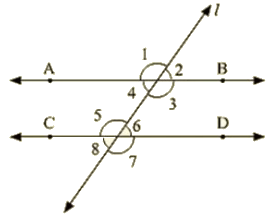Ans :     Let ∠1 = 3x and ∠2 = 2x

∠1 and ∠2 are linear pair of angle

Now, ∠1 and ∠2

⇒  3x + 2x = 180

⇒ 5x  = 180

⇒ x = 180 / 5

⇒ x = 36

∠1 = 3x = 108,∠2 = 2x = 72

We know, Vertically opposite angles are equal

∠1=∠3 = 108

∠2 =∠4 = 72

∠6 =∠7=108

∠5 =∠8 = 72

We also know, corresponding angles are equal

∠1=∠5 = 108

∠2 = ∠6 = 72

Q 2: In the below fig, I, m and n are parallel lines intersected by transversal p at X. Y and Z respectively. Find ∠1,∠2 and ∠3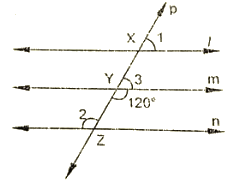Ans : From the given figure :

∠3+∠mYZ = 180  [Linear pair]

⇒ ∠3 =180–120

⇒ ∠3 = 60

Now line I parallel to m

∠1 =∠3 [Corresponding angles]

∠1 = 60

Also m parallel to n

⇒ ∠2 = 120  [Alternative interior angle]

Hence, ∠1 =∠3 = 60

∠2 = 120

3. In the below fig, AB || CD || EF and GH || KL Find ∠HKL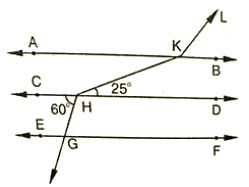Ans  :    Produce LK to meet GF at N.

Now, alternative angles are equal

∠CHG = ∠HGN = 60∘

∠HGN = ∠KNF = 60∘        [Corresponding angles]

Hence, ∠KNG = 180–60 = 120

⇒ ∠GNK =∠AKL = 120 [Corresponding angles]

∠AKH =∠KHD = 25        [alternative angles]

Therefore, ∠HKL=∠AKH+∠AKL = 25+120 = 145

Q 4 : In the below fig, show that AB || EF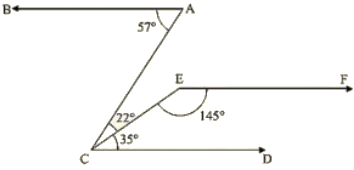Ans : Produce EF to intersect AC at K.

Now, ∠DCE+∠CEF = 35+145 = 180∘

Therefore, EF || CD       (Since Sum of Co-interior angles is 180) —–(1)

Now, ∠BAC =∠ACD = 57

⇒ BA || EF               [Alternative angles are equal]        —–(2)

From (1) and (2)

AB || EF [Since, Lines parallel to the same line are parallel to each other]

Hence proved.

Q 5 : If below fig.if AB || CD and CD || EF, find ∠ACE.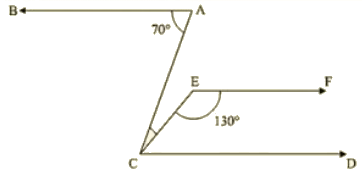Ans :  Since EF || CD

Therefore,  EFC + ECD = 180       [co-interior angles are supplementary]

⇒ ECD = 180 – 130 = 50

Also BA || CD

⇒ BAC = ACD = 70                [alternative angles]

But, ACE + ECD =70

⇒ ACE = 70 — 50 = 20

Q 6 : In the below fig, PQ || AB and PR || BC. If ∠QPR = 102, determine ∠ABC Give reasons.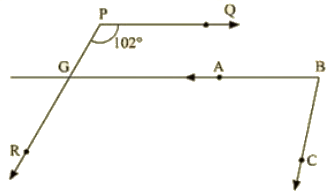Ans :  AB is produce to meet PR at K

Since PQ || AB

∠QPR = ∠BKR = 102              [corresponding angles]

Since PR || BC

∠RKB+∠CBK = 180               [ Since Corresponding angles are supplementary]

∠CKB=180–102 = 78

∴ ∠CKB = 78°

Q 7 : In the below fig, state Which lines are parallel and why?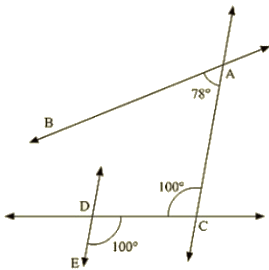Ans :  Vertically opposite angles are equal

⇒ ∠EOC = ∠DOK = 100∘

∠DOK = ∠ACO = 100∘

Here two lines EK and CA cut by a third line and the corresponding angles to it are equal Therefore, EK || AC.

8. In the below fig. if l||m, n || p and ∠1 = 85°. find ∠2.Ans :  Corresponding angles are equal

⇒ ∠1=∠3 = 85

By using the property of co-interior angles are supplementary

∠2+∠3 = 180

∠2+55 = 180

∠2 = 180–85

∠2 = 95

Q 9 : If two straight lines are perpendicular to the same line, prove that they are parallel to each other.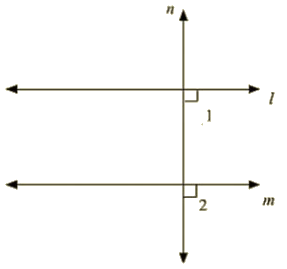Ans : Given m perpendicular t and l perpendicular to t

∠1=∠2 = 90

Since,  I and m are two lines and it is transversal and the corresponding angles are equal

L || M

Hence proved

Q 10 : Prove that if the two arms of an angle are perpendicular to the two arms of another angle. then the angles are either equal or supplementary.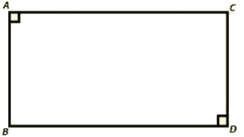Ans : Consider be angles AOB and ACB

Given  0A perpendicular to A0, also 0B perpendicular to BO

To prove : ∠AOB+∠ACB=180∘ (or) ∠AOB+∠ACB = 180

Proof :  In a quadrilateral = ∠A+∠O+∠B+∠C = 360

[ Sum of angles of quadrilateral is 360 ]

⇒ 180+ O +B + C = 360

⇒ O+ C = 360 –180

Hence AOB + ACB = 180                     —–(1)

Also, B + ACB = 180

⇒ ACB =180 – 90 = ACES = 90°        —–(2)

From (i) and (ii), ACB = A0B = 90

Hence, the angles are equal as well as supplementary.

Q 11 : In the below fig, lines AB and CD are parallel and P is any point as shown in the figure. Show that ∠ABP+∠CDP = ∠DPB.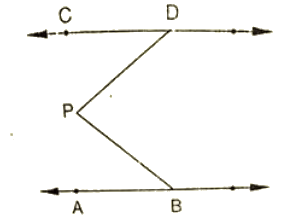Ans :

Given that AB ||CD

Let EF be the parallel line to AB and CD which passes through P

It can be seen from the figure

Alternative angles are equal

∠ABP = ∠BPF

Alternative angles are equal

∠CDP = ∠DPF

∠ABP+∠CDP = ∠BPF+∠DPF

∠ABP+∠CDP = ∠DPB

Hence proved

AB parallel to CD, P is any point

To prove: ∠ABP+∠BPD+∠CDP = 360

Construction : Draw EF || AB passing through P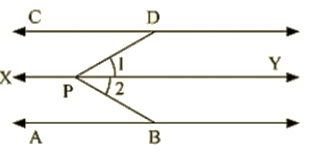Proof : Since AB  ||EF and AB || CD, Therefore EF || CD                     [Lines parallel to the same line are parallel to each other)

∠ABP+∠EPB = 180      [Sum of co-interior angles is180)

∠EPD+∠COP = 180       —–(1) [Sum of co-interior angles is180)

∠EPD+∠CDP = 180       —–(2)

∠ABP+∠EPB+∠EPD+∠CDP = (180+180)

∠ABP+∠EPB+∠COP = 360

Q 12 : In the below fig, AB || CD and P is any point shown in the figure. Prove that : ∠ABP+∠BPD+∠CDP = 360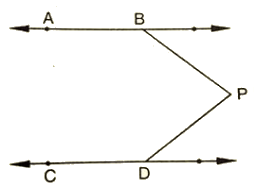Ans : Through P, draw a line PM parallel to AB or CD.

Now,

A8 || PM ⇒ ABP + BPM = 180

And

CD||PM = MPD + CDP = 180

Adding (i) and (ii), we get A8P + (BPM + MPD) CDP = 360

⇒ ABP + BPD + CDP = 360

Q 13 : Two unequal angles of a parallelogram are in the ratio 2 : 3. Find all its angles in degrees.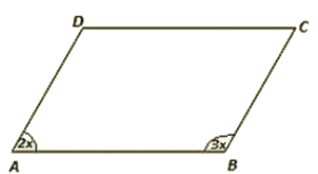Ans : Let A = 2x and B = 3x

Now, A +B = 180 [Co-interior angles are supplementary]

2x  + 3x – 180 [AD II BC and AB is the transversal)

⇒ 5x = 180

x = 180/5

x = 36

Therefore, A = 2 x 36 = 72

b = 3 x 36  = 108

Now, A = C = 72  [Opposite side angles of a parallelogram are equal)

B = D = 108

Q 14 :  If each of the two lines is perpendicular to the same line, what kind of lines are they to each other?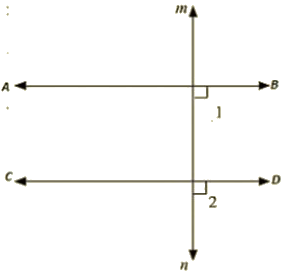Ans :

Let AB and CD be perpendicular to MN

ABD =  90 [AB perpendicular to MN]             —– (i)

CON = 90 [CO perpendicular to MN]             —– (ii)

Now, ABD = CDN = 90   (From (i) and (ii))

AB parallel to CD,

Since corresponding angles are equal

Offer running on EduRev: Apply code STAYHOME200 to get INR 200 off on our premium plan EduRev Infinity!

91 docs

,

,

,

,

,

,

,

,

,

,

,

,

,

,

,

,

,

,

,

,

,

,

,

,

,

,

,

,

,

,

,

,

,

;